• 常见的广义线性模型有：probit模型、poisson模型、对数线性模型等等。对数线性模型里有：logistic regression、Maxinum entropy。本篇是对逻辑回归理论和应用的学习总结、以及广义线性模型导出逻辑回归的过程，下一...
        经典线性模型自变量的线性预测就是因变量的估计值。 广义线性模型：自变量的线性预测的函数是因变量的估计值。常见的广义线性模型有：probit模型、poisson模型、对数线性模型等等。对数线性模型里有：logistic regression、Maxinum entropy。本篇是对逻辑回归的学习总结，以及广义线性模型导出逻辑回归的过程。下一篇将是对最大熵模型的学习总结。本篇介绍的大纲如下：
1、逻辑斯蒂分布，logit转换
2、在二分类问题中，为什么弃用传统的线性回归模型，改用逻辑斯蒂回归？
3、逻辑回归模型的求解过程？
4、实际应用逻辑回归时数据预处理的经验总结。但经验有限，如果有哪位网友这块经验丰富，忘指教，先谢过
5、为什么我们在实际中，经典线性模型的优化目标函数是最小二乘，而逻辑回归则是似然函数
6、从最根本的广义线性模型角度，导出经典线性模型以及逻辑回归

1、逻辑斯蒂分布，logit转换
一个连续随机变量X，如果它的分布函数形式如下，则X服从逻辑斯蒂分布，F（x）的值在0~1之间，它的的图形是一条S型曲线。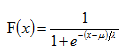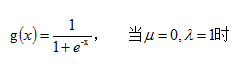2、在二分类问题中，为什么弃用传统的线性回归模型，改用逻辑斯蒂回归？
线性回归用于二分类时，首先想到下面这种形式，p是属于类别的概率：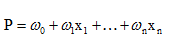但是这时存在的问题是：
1）等式两边的取值范围不同，右边是负无穷到正无穷，左边是[0,1]，这个分类模型的存在问题
2）实际中的很多问题，都是当x很小或很大时，对于因变量P的影响很小，当x达到中间某个阈值时，影响很大。即实际中很多问题，概率P与自变量并不是直线关系。
所以，上面这分类模型需要修整，怎么修正呢？统计学家们找到的一种方法是通过logit变换对因变量加以变换，具体如下：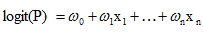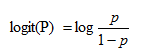从而，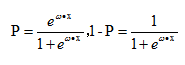这里的P完全解决了上面的两个问题。
3、逻辑回归模型的求解过程？
1）求解方式
逻辑回归中，Y服从二项分布，误差服从二项分布，而非高斯分布，所以不能用最小二乘进行模型参数估计，可以用极大似然估计来进行参数估计。
2）似然函数、目标函数
严谨一点的公式如下：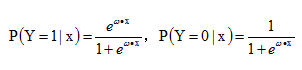似然函数如下：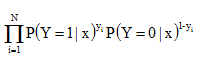对数似然函数，优化目标函数如下：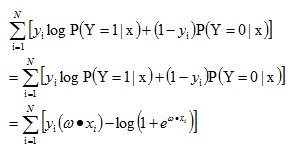整个逻辑回归问题就转化为求解目标函数，即对数似然函数的极大值的问题，即最优化问题，可采用梯度下降法、拟牛顿法等等。

4、实际应用逻辑回归时数据预处理的经验总结，但经验有限，如果有哪位网友这块经验丰富，忘指教，先谢过
1）枚举型的特征直接进行binary
2）数值型特征，可以：标准化、根据分布进行binary
3）进行pairwise
5、为什么我们在实际中，经典线性模型的优化目标函数是最小二乘，而逻辑回归则是似然函数
下面公式直接从Ng notes里面复制过来。
1） 经典线性模型的满足下面等式：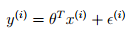这里有个假设，即最后这个误差扰动项独立同分布于均值为0的正态分布，即：从而：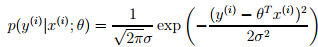由于有上面的假设，从而就有下面的似然函数：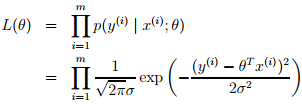从而这线性回归的问题就可转化为最大化下面的对数似然估计，由于下面公式前面的项是常数，所以这个问题等价于最小化下面等式中的最后一项，即least mean squares。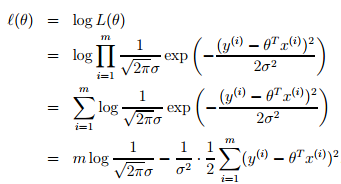2）逻辑斯蒂回归中，因变量y不再是连续的变量，而是二值的{0,1}，中间用到logit变换，将连续性的y值通过此变换映射到比较合理的0~1区间。在广义线性回归用于分类问题中，也有一个假设（对应于上面回归问题中误差项独立同分布于正态分布），其中h(x)是logistic function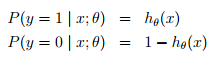即，给定x和参数，y服从二项分布，上面回归问题中，给定x和参数，y服从正态分布。从而。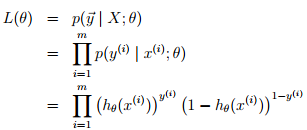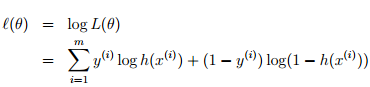问题不同（一个是分类、一个是回归）对应假设也就不同，决定了logistic regression问题最优化目标函数是上面这项，而非回归问题中的均方误差LMS。
6、从最根本的广义线性模型角度，导出经典线性模型以及逻辑回归
1）指数家族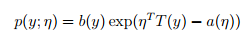当固定T时，这个分布属于指数家族中的哪种分布就由a和b两个函数决定。下面这种是伯努利分布，对应于逻辑回归问题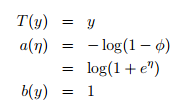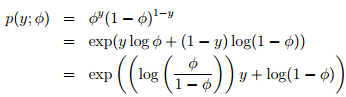注：从上面可知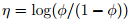，从而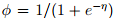，在后面用GLM导logistic
regression的时候会用到这个sigmoid函数。

下面这种是高斯分布，对应于经典线性回归问题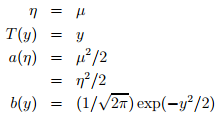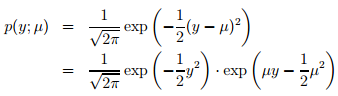2）GLM（广义线性模型）
指数家族的问题可以通过广义线性模型来解决。如何构建GLM呢？在给定x和参数后，y的条件概率p(y|x,θ) 需要满足下面三个假设：
assum1)      y | x; θ ∼ ExponentialFamily(η).
assum2)      h(x) = E[y|x]. 即给定x，目标是预测T(y)的期望，通常问题中T(y)=y
assum3)       η = θTx，即η和x之间是线性的
3）经典线性回归、逻辑回归
经典线性回归：预测值y是连续的，假设给定x和参数，y的概率分布服从高斯分布（对应构建GLM的第一条假设）。由上面高斯分布和指数家族分布的对应关系可知，η=µ，根据构建GLM的第2、3条假设可将model表示成：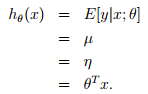逻辑回归：以二分类为例，预测值y是二值的{1,0}，假设给定x和参数，y的概率分布服从伯努利分布（对应构建GLM的第一条假设）。由上面高斯分布和指数家族分布的对应关系可知，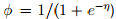，根据构建GLM的第2、3条假设可model表示成：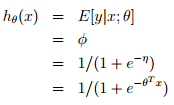可以从GLM这种角度理解为什么logistic regression的公式是这个形式~

参考资料：
 NG的lecture notes，http://cs229.stanford.edu/notes/cs229-notes1.pdf
 其他网络资源

展开全文逻辑回归 广义线性模型
• 中科大对数线性模型读书笔记，数据预处理的使用场景。
• 对数线性模型log linear model 对数线性模型有：最大熵模型和逻辑斯谛回归。 特征和指示特征 对数线性模型的一般形式 [概率图模型原理与技术] 某小皮 对数线性模型的不同形式 因子图 将因子...
http://blog.csdn.net/pipisorry/article/details/52788947

对数线性模型log linear model

对数线性模型有：最大熵模型和逻辑斯谛回归。

特征和指示特征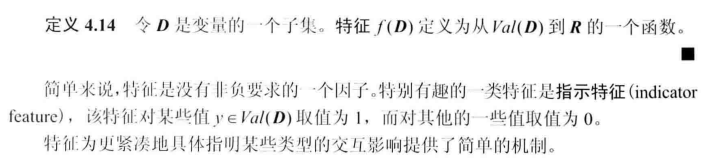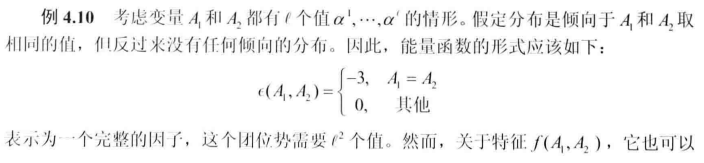对数线性模型的一般形式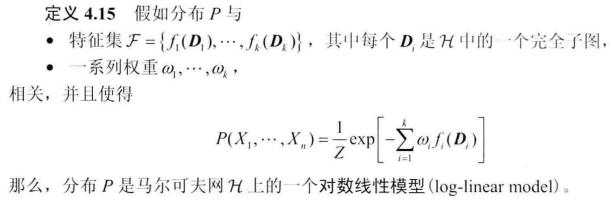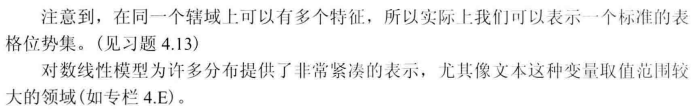[概率图模型原理与技术]

某小皮

对数线性模型的不同形式

因子图

将因子转换到对数空间，成为对数线性模型。

[PGM：无向图模型：马尔可夫网 ：对数线性模型]

最大熵模型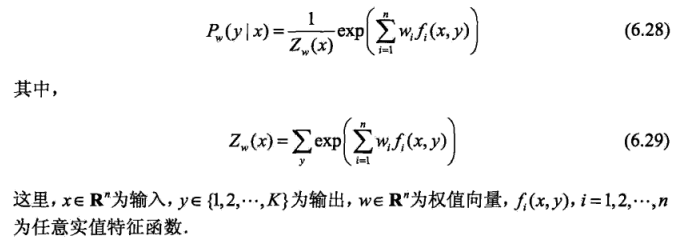[最大熵模型The Maximum Entropy：模型] [最大熵模型：学习]

逻辑斯谛回归Logistic Regression

多类分类的LR模型生成的推导：（两类分类更简单，直接类比嘛）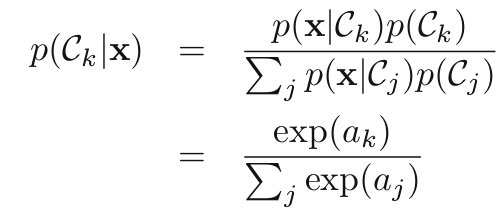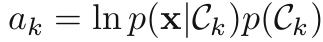lz：这里ak是对数表示的，而给定类条件概率密度p(x|ck)如高斯分布时，ak是通常是线性表示的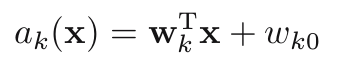，所以才叫对数线性模型吧。

因为ak通常可以使用线性表示，所以多类LR模型使用判别式直接定义成：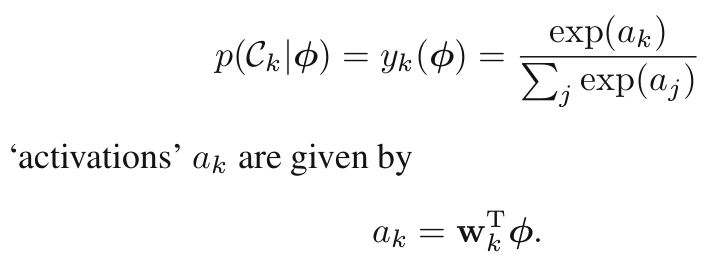lz: 就是把一般形式中的feature特征fi(Di)定义为ak了。

LR模型的导出

lz也不知道LR模型怎么来的，不过lz总结了几种都可以解释的方面：

1 回归模型+logistic函数直接得到

2 最大熵模型的特例，即直接将特征f(x, y)设为X=x（即在所有X=x的值上搞一个权重w）。

3 广义线性模型导出[对数线性模型之一(逻辑回归), 广义线性模型学习总结]

4 生成式模型+高斯形式的类条件概率分布得到

from: http://blog.csdn.net/pipisorry/article/details/52788947

ref:


展开全文• 从线性到非线性模型 1、线性回归，岭回归，Lasso回归，局部加权线性回归 2、logistic回归，softmax回归，最大熵模型 3、广义线性模型 4、Fisher线性判别和线性感知机 5、三层...
从线性到非线性模型
1、线性回归，岭回归，Lasso回归，局部加权线性回归
2、logistic回归，softmax回归，最大熵模型
3、广义线性模型
4、Fisher线性判别和线性感知机
5、三层神经网络
6、支持向量机
code: https://github.com/myazi/myLearn
二、Logistic回归和SoftMax回归，最大熵模型
一、Logistic回归
分类问题可以看作是在回归函数上的一个分类。一般情况下定义二值函数，然而二值函数不易优化，一般采用sigmoid函数平滑拟合（当然也可以看作是一种软划分，概率划分）：从函数图像我们能看出，该函数有很好的特性，适合二分类问题。至于为何选择Sigmoid函数，后面可以从广义线性模型推导出Sigmoid函数。
逻辑回归可以看作是在线性回归的基础上构建的分类模型，理解的角度有多种，最直接的理解是考虑逻辑回归是将线性回归值离散化。即一个二分类问题如下：（二值函数）
KaTeX parse error: No such environment: equation at position 8:
\begin{̲e̲q̲u̲a̲t̲i̲o̲n̲}̲
\begin{split}…
sigmoid函数
$g(z)=\frac{1}{1+e^{-z}},g^{‘}(z)=g(z)(1-g(z))$二分类问题属于一种硬划分，即是与否的划分，而sigmoid函数则将这种硬划分软化，以一定的概率属于某一类（且属于两类的加和为1）。Sigmoid函数将线性回归值映射到 $[0,1]$区间，从函数图像我们能看出，该函数有很好的特性，适合二分类问题。 因此逻辑回归模型如下：
KaTeX parse error: No such environment: equation at position 8:
\begin{̲e̲q̲u̲a̲t̲i̲o̲n̲}̲
\begin{split}…
这里对于目标函数的构建不再是最小化函数值与真实值的平方误差了，按分类原则来讲最直接的损失因该是0-1损失，即分类正确没有损失，分类错误损失计数加1。但是0-1损失难以优化，存在弊端。结合sigmoid函数将硬划分转化为概率划分的特点，采用概率$h_{\theta}(x^{(i)})$的对数损失（概率解释-N次伯努利分布加最大似然估计），其目标函数如下：
KaTeX parse error: No such environment: equation at position 8:
\begin{̲e̲q̲u̲a̲t̲i̲o̲n̲}̲
\begin{split}
…
同样采用梯度下降的方法有：
KaTeX parse error: No such environment: equation at position 8:
\begin{̲e̲q̲u̲a̲t̲i̲o̲n̲}̲
\begin{split}
…
又：
KaTeX parse error: No such environment: equation at position 8:
\begin{̲e̲q̲u̲a̲t̲i̲o̲n̲}̲
\begin{split}
…
所以有：
$\theta_{j}=\theta_{j}-\alpha \left(y^{(i)}-h_{\theta}(x^{(i)})\right)x$
概率解释
逻辑回归的概率解释同线性回归模型一致，只是假设不再是服从高斯分布，而是$p\left ( y|x;\theta \right )$服从0-1分布，由于 ，假设随机变量y服从伯努利分布是合理的 。即：
KaTeX parse error: No such environment: equation at position 8:
\begin{̲e̲q̲u̲a̲t̲i̲o̲n̲}̲
\begin{split}
…
所以最大化似然估计有：
KaTeX parse error: No such environment: equation at position 8:
\begin{̲e̲q̲u̲a̲t̲i̲o̲n̲}̲
\begin{split}
…
logistic采用对数损失原因
采用对数损失的原因有二：
​	1)从概率解释来看，多次伯努利分布是指数的形式。由于最大似然估计导出的结果是概率连乘，而概率（sigmoid函数）恒小于1，为了防止计算下溢，取对数将连乘转换成连加的形式，而且目标函数和对数函数具备单调性，取对数不会影响目标函数的优化值。
​	2）从对数损失目标函数来看，取对数之后在求导过程会大大简化计算量。
二、SoftMax回归
Softmax回归可以看作是Logistic回归在多分类上的一个推广。考虑二分类的另一种表示形式：
$\left [ k_{1},1-k_{1} \right ]\rightarrow \begin{bmatrix} k_{1}\\ k_{2} \end{bmatrix}$
当logistic回归采用二维表示的话，那么其损失函数如下：
KaTeX parse error: No such environment: equation at position 8:
\begin{̲e̲q̲u̲a̲t̲i̲o̲n̲}̲
\begin{split}
…
其中，在逻辑回归中两类分别为$k_{1}$，$1-k_{1}$,二在softmax中采用$k_{1}，$$k_{2}$两个随机变量组成二维向量表示，当然隐含约束$k_{1}+k_{2}=1$.为了更好的表示多分类问题，将$y\in \left \{ 1,2,..K \right \}$（不一定理解为y的取值为k，更应该理解为y可以取k类）多分类问题进行如下表示。
$T(k)=\begin{bmatrix} 0\\ 0\\ .\\ 1\\ .\\ 0 \end{bmatrix}$
其中向量的第k位为1，其他位为0，也就是当$y=k$ 时将其映射成向量时对应第k位为1。采用多维表示之后，那么对于每一维就变成了一个单独的二分类问题了，所以softmax函数形式如下：
$h_{\theta}(x^{(i)})=\frac{1}{\sum_{ k=1}^{K}exp\left ( \theta _{k}^{T}x^{(i)} \right )}\begin{bmatrix} exp\left ( \theta _{k}^{T}x^{(i)} \right )\\ exp\left ( \theta _{k}^{T}x^{(i)} \right )\\ .\\ exp\left ( \theta _{k}^{T}x^{(i)} \right ) \end{bmatrix}$
其中函数值是一个$K$维的向量，同样采用对数损失（多项式分布和最大似然估计），目标函数形式是logistic回归的多维形式。
KaTeX parse error: No such environment: equation at position 8:
\begin{̲e̲q̲u̲a̲t̲i̲o̲n̲}̲
\begin{split}
…
其中$y^{ik}$表示第$i$个样本的标签向量化后第k维的取值0或者1.可以看出Softmax的损失是对每一类计算其概率的对数损失，而logistic回归是计算两类的回归，其本质是一样。Logistic回归和Softmax回归都是基于线性回归的分类模型，两者无本质区别，都是从二项分布和多项式分布结合最大对数似然估计。只是Logistic回归常用于二分类，而Softmax回归常用于多分类。而且Logistic回归在考虑多分类时只考虑n-1类。
概率解释
​	二分类与多分类可以看作是N次伯努利分布的二项分布到多项分布的一个推广，概率解释同Logistic回归一致。详细解释放到广义线性模型中。
二分类转多分类思想
对于多分类问题，同样可以借鉴二分类学习方法，在二分类学习基础上采用一些策略以实现多分类，基本思路是“拆解法”，假设N个类别$C_{1},C_{2},.C_{i}.,C_{n}$，经典的拆分算法有“一对一”，“一对多”，“多对多”，
一对一的基本思想是从所有类别中选出两类来实现一个两分类学习器，即学习出$C_{N}^{2}=N(N-1)/2$个二分类器，然后对新样本进行预测时，对这 $C_{N}^{2}$个分类器进行投票最终决定属于那一类。
一对多的基本思想是把所有类别进行二分类，即属于$C_{i}$类和非$C_{i}$两类，这样我们就需要N个分类器，然后对新样本进行预测时，与每一个分类器比较，最终决定属于哪一类。这其实就是Softmax的思想，也是SVM多分类的思想。
//多对多的基本思想是
三、最大熵模型
​	很奇怪，为什么会把最大熵模型放到这，原因很简单，它和Logistic回归和SoftMax回归实在是惊人的相似，同属于对数线性模型。
熵的概念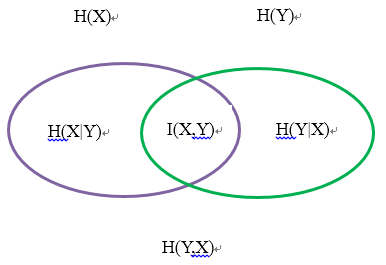信息熵：熵是一种对随机变量不确定性的度量，不确定性越大，熵越大。若随机变量退化成定值，熵为0。均匀分布是“最不确定”的分布 。
假设离散随机变量X的概率分布为$P(X)$，则其熵为：
$H(X)=-\sum_{x}P(x)logP(x)$
其中熵满足不等式$0\leq H(P) \leq log|X|$。
联合熵：对于多个随机变量的不确定性可以用联合熵度量
假设离散随机变量$X,Y$的联合概率分布为$P(X,Y)$，则其熵为：
$H(X,Y)=-\sum_{x}\sum_{y}P(x,y)logP(x,y)$
条件熵：在给定条件下描述随机变量的不确定性
假设离散随机变量$X,Y$，在给定$Y$的条件下$X$的不确定性为条件熵H(X|Y)，也就等于$H(X,Y)-H(Y)$
$H(X|Y)=-\sum_{x,y}P(x,y)log(P(x|y))$
互信息：衡量两个随机变量相关性的大小$I(X,Y)=H(X)+H(Y)-H(X,Y)$
$I(X,Y)=-\sum_{x,y}P(x,y)log\frac{P(x,y)}{P(x)P(y)}$
相对熵（KL散度）：衡量对于同一个随机变量两个概率分布$p(x),q(x)$的差异性
$D(p||q)=\sum_{x}p(x)log\frac{p(x)}{q(x)}=E_{p(x)}log\frac{p(x)}{q(x)}$
有互信息和相对熵的定义有下式：
$I(X,Y)=D(P(X,Y)||P(X)P(Y))$
关于熵的介绍就到此，不细究，虽然上面的这些定义在机器学习中都会遇到，不过后面涉及到的主要还是熵和条件熵。
最大熵模型
最大熵原理是概率模型学习中的一个准则。最大熵原理认为，学习概率模型时，在所有可能的概率模型分布中（满足所有条件下），熵最大的模型是最好的模型。熵最大即为最均匀的分布，从某种角度讲 均匀分布总是符合我们理解的损失风险最小，也就是“不要不所有的鸡蛋放到一个篮子里，均匀的放置”。
给定训练集$T=\{ (x_{1},y_{1}),(x_{2},y_{2})..(x_{m},y_{m})\}$，假设$X \in \chi \subseteq R^{n}$表示输入，$y\in \phi$表示输出，分类模型是一个以条件概率分布$P(Y|X)$输出$Y$，也就是说在满足条件的所有可能集中，条件熵$P(Y|X)$最大的模型即为最好的模型。其中条件为隐藏在数据的期望。
一般来讲，最大熵模型常用于处理离散化数据集，定义随机变量$X,Y$的特征模板，从数据中统计他们的期望作为最大熵模型的条件
特征函数：
$f(x,y)=\left\{\begin{matrix} 1，x，y满足某一事实\\ 0，否则 \end{matrix}\right.$
约束条件：对于任意的特征函数$f$，我们可以统计其在数据中的经验分布$\widetilde{P}(x,y)$的期望
$E_{\widetilde{p}}(f)=\sum_{x,y}\widetilde{P}(x,y)f(x,y)$
特征函数$f$关于模型$P(Y|X)$和先验$\widetilde{P}(X)$的期望
$E_{p}(f)=\sum_{x,y}\widetilde{P}(x)P(y|x)f(x,y)$
所以，满足约束条件的模型集合为：
$\Omega \equiv \{ P\in \boldsymbol{P}| E_{p}(f_{i})=E_{\widetilde{p}}(f_{i}),i=1..n\}$
因此最大熵模型的形式化表示如下：
KaTeX parse error: No such environment: equation at position 8:
\begin{̲e̲q̲u̲a̲t̲i̲o̲n̲}̲
\begin{split}…
由拉格让日乘子法，引入拉格让日乘子，定义拉格让日函数：
KaTeX parse error: No such environment: equation at position 8:
\begin{̲e̲q̲u̲a̲t̲i̲o̲n̲}̲
\begin{split}
…
根据拉格朗日乘子法，$L(P) \geq L(P,w)$,当且仅当满足拉格朗日乘子法的所有必要条件等式成立，原问题也就是一个最小化最大问题
$\min_{P \in C}\max_{w}L(P,w)$
对偶问题是：
$\max_{w} \min_{P \in C}L(P,w)$
求解对偶问题，第一步最小化内部$\min_{P \in C}L(P,w)$，$\min_{P \in C}L(P,w)$是我们需要求解的模型，也是关于$w$的函数。将模型的最优解记为$P_{w}$：
$P_{w}=arg\max_{P \in C}L(P,w)=P_{w}(y|x)$
那么外层最大化目标函数为：
$\max_{w}\Phi(w)\\ \Phi(w)=\min_{p \in C}L(P,w)=L(P_{w},w)$
为了求解$P_{w}(y|x)$，根据KKT条件对$P(y|x)$求偏导：
KaTeX parse error: No such environment: equation at position 8:
\begin{̲e̲q̲u̲a̲t̲i̲o̲n̲}̲
\begin{split}
…
求解得：
$P(y|x)=exp\left( \sum_{i} w_{i}f_{i}(x,y) +w_{0}-1 \right)=\frac{ \left(exp \sum_{i} w_{i}f_{i}(x,y)\right)}{exp(1-w_{0})}$
这里，虽然我们不知道$w_{0}$，但是由于$\sum_{y}P(y|x)=1$,所以分母一定是对$y$的所有可能的归一化因子
$P_{w}(y|x)=\frac{1}{z_{w}(x)} \left(exp \sum_{i} w_{i}f_{i}(x,y)\right)\\ z_{w}(x)=\sum_{y}exp(\sum_{i}w_{i}f_{i}(x,y))$
到此，内部最小化求解得到了$P_{w}(y|x)$，回到外部目标$\max_{w}\Phi(w)$，将$P_{w}(y|x)$代回拉格朗日函数有：
\begin{aligned} \Phi(w) &=\sum_{x,y}\widetilde{P}(x)P_w(y|x)logP_w(y|x) + \sum^n_{i=1}w_i\left (\sum_{x,y}\widetilde{P}(x ,y)f(x,y) -\sum_{x,y}\widetilde{P}(x)P_w(y|x)f(x,y) \right )\\ &= \sum_{x,y} \widetilde{P}(x,y)\sum_{i=1}^nw_if_i(x,y) +\sum_{x,y}\widetilde{P}(x)P_w(y|x)\left (logP_w(y|x) - \sum_{i=1}^nw_if_i(x,y) \right) \\ &=\sum_{x,y} \widetilde{P}(x,y)\sum_{i=1}^nw_if_i(x,y) +\sum_{x,y}\widetilde{P}(x)P_w(y|x)logZ_w(x)\\ &=\sum_{x,y} \widetilde{P}(x,y)\sum_{i=1}^nw_if_i(x,y) +\sum_x\widetilde{P}(x)logZ_w(x)\sum_yP_w(y|x)\\ &=\sum_{x,y} \widetilde{P}(x,y)\sum_{i=1}^nw_if_i(x,y) +\sum_x\widetilde{P}(x)logZ_w(x)\\ \end{aligned}
因此，$\max_{w} \Phi(w)$的最优解为：
$w^* = arg \max_w \Phi(w)$
代回$P_{w}(y|x)$，我们可以得到最终的分类模型，同样我们发现最大熵模型也是一个对数线性模型。
概率解释：
已知训练集的经验概率分布$\widetilde{P}(x,y)$，条件概率分布$P(y|x)$的对数似然函数为：
$L_{\widetilde{P}}(P_w) = log\prod_{x,y}P(y|x)^{\widetilde{P}(x,y)} = \sum_{x,y}\widetilde{P}(x,y)logP(y|x)$
其中，我们发现对数似然函数与条件熵的形式一致，最大熵模型目标函数前面有负号（这与最大化对数似然函数完全相反），同时最大熵模型中有约束条件。也正是因为约束条件，我们将原问题转化为对偶问题后发现，在满足约束条件的对偶函数的极大化等价于最大化对数似然函数。
当条件概率$P(y|x)$满足约束条件，在对偶问题求解过程中我们有：
$P_{w}(y|x)=\frac{1}{z_{w}(x)} \left(exp \sum_{i} w_{i}f_{i}(x,y)\right)\\ z_{w}(x)=\sum_{y}exp(\sum_{i}w_{i}f_{i}(x,y))$
代入到对数似然函数，同样有：
\begin{aligned} L_{\widetilde{P}}(P_w) &= \sum_{x,y}\widetilde{P}(x,y)logP(y|x)\\ &= \sum_{x,y}\widetilde{P}(x,y)\left ( \sum_{i=1}^n w_if_i(x,y) -logZ_w(x)\right )\\ &= \sum_{x,y}\widetilde{P}(x,y)\sum_{i=1}^n w_if_i(x,y) - \sum_{x,y}\widetilde{P}(x,y)logZ_w(x)\\ &= \sum_{x,y}\widetilde{P}(x,y)\sum_{i=1}^n w_if_i(x,y) - \sum_{x}\widetilde{P}(x)logZ_w(x)\\ \end{aligned}
最后，我们再来看对偶函数表达式，我们发现，第一项其实是$X,Y$的联合熵$H(X,Y)$，第二项是X的信息熵$H(X)$，回看熵的示意图，我们发现，我们最大化的目标还是条件熵$H(Y|X)$。下面再来对比下Logistic回归，SoftMax回归，最大熵模型
1）同属于对数线性模型
2）Logistic回归和SoftMax回归都基于条件概率$P(y|x)$满足一个伯努利分布，N重伯努利分布，而最大熵模型以期望为准，没有该假设
3）由于都采用线性模型，三者都假设特征之间是独立的
最大熵模型的优化问题
最大熵模型从拉格朗日乘子法最大化对偶函数，还是从最大化对数似然函数，其目标函数如下：
$L_{\widetilde{P}}(P_w)= \sum_{x,y}\widetilde{P}(x,y)\sum_{i=1}^n w_if_i(x,y) - \sum_{x}\widetilde{P}(x)logZ_w(x)\\$
常用的梯度优化算法都可以，另外对于最大熵模型也有专门的算法有GIS IIS 算法 。


展开全文• 对数线性模型包括逻辑回归、最大熵模型和条件随机场等 1、模型 条件概率分布（对数线性模型、概率模型）、判别模型 逻辑回归： 概率分布可由广义线性模型推导得到 多分类时，分子部分为：第k类exp(-wk*x+b)，...
建议首先看cs229讲的广义线性模型、exponential family（指数分布族）
对数线性模型包括逻辑回归、最大熵模型和条件随机场等
1、模型
条件概率分布（对数线性模型、概率模型）、判别模型逻辑回归：
概率分布可由广义线性模型推导得到
多分类时，分子部分为：第k类exp(-wk*x+b)，第一类为1
分母部分为所有类分子之和，即标准化因子
最大熵模型：
概率分布由最大熵原理进行建模
分子部分为：exp(特征函数的加权和）
分母部分为对分子的标准化因子
条件随机场：
概率分布由线性链（简单情况）条件随机场确定
分子为：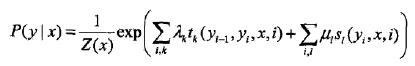分母为：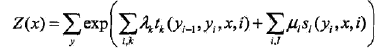2、策略
最大似然估计
注：凡是已知条件概率分布（其实是似然）的模型，一般策略都是最大似然估计，即最小化对数损失函数
最大似然估计是一种参数估计方法，其他还有最大后验概率（考虑先验的最大似然）、贝叶斯估计（最大后验的基础上进一步增强，分母看做归一化因子，即对分子的积分）最小二乘估计（最小平方估计，最小化误差的平方）等，会在后面总结一节
3、算法
对数线性模型参数估计用到的算法即是最大似然估计用的算法，也就是梯度下降法等
注：条件随机场其他两个问题中，概率用前向-后向算法，预测用维特比算法（同隐马尔科夫模型）
4、补充
最大熵模型推导过程：
一是最大化条件熵
条件熵等于联合熵减去熵
二是由特征函数确定约束条件
特征函数关于（联合概率的经验分布）的期望值等于特征函数关于条件分布（模型）与边缘概率的期望值
三是建立原始约束最优化问题
即max最大熵模型
s.t 约束条件
四是建立最小最大化原问题
引入拉格朗日乘子
五是转化为最大最小化问题
引入kkt条件
六是转化为极大似然估计
展开全文•  经典线性模型自变量的线性预测就是因变量的估计值。 广义线性模型：自变量的线性预测的函数是因变量的估计值。常见的广义线性模型有：probit模型、poisson...对数线性模型里有：logistic regression、Maxi
• http://blog.csdn.net/pipisorry/article/details/52788947 特征和指示特征 对数线性模型log linear model 对数线性模型有：最大熵模型和逻辑斯谛回归。 [概率图模型原理与技术] [PGM：无向图...
• MIT自然语言处理第五讲：最大熵和对数线性模型（第一部分） 自然语言处理：最大熵和对数线性模型 Natural Language Processing: Maximum Entropy and Log-linear Models  作者：Regina Barzilay...MIT 自然语言处理 NLP
• 对数线性模型》是最好的对数线性模型教学材料之一，不仅讨论了一般对数线性模型，还讨论了logit模型，这一模型通过分析作为自变量函数的因变量的期望发生比来检验自变量与因变量之间的关系。作者从处理二分变量的...
• 对数线性模型是无向图中经常使用的一种模型。其利用特征函数以及参数的方式对势函数进行定义，可获得较好的效果。在之前有向图的学习中，我们发现可以利用d-seperet，充分统计，狄利克雷函数等方式来很优雅的获得...
• 二项Logistic回归模型由条件概率分布P(Y|X)表示，X为随机变量，取值为实数，Y同为随机变量，但取值为1或0； 二项 Logistic回归模型的条件概率分布： 其中，w称为权值向量，b为偏置，x为输入，Y为输出...
• 对于列联表资料，通常作χ2 检验，但χ2 检验无法系统地评价变量间的联系，也无法估计变量间相互作用的大小，而对数线性模型是处理这些问题的最佳方法。 see more ...attributes less each 工具
• 常用的三个线性模型的原理及python实现——线性回归（Linear Regression）、对数几率回归（Logostic Regression）、线性判别分析（Linear Discriminant）。 这可能会是对线性模型介绍最全面的博客一、线性模型 ...python lda算法原理
• 第4-18章主要介绍各种统计分析方法及其对应SPSS过程的操作方式，包括描述性统计、均值比较、一般线性模型、相关分析、回归分析、对数线性模型、聚类分析、生存分析、时间序列分析、多重响应分析等几大类。...
• 线性模型基本形式：f(x)=w1*x1+w2*x2+w3*x3+...+wd*xd+b 向量形式：f(x)=w'x+b(w'指w转置w'=(w1,w2,w3,...,wd)) 回归任务最常用均方误差作为性能度量，见下图 ...如对数线性回归：lny=w'x+b，让e^(w'x+b)逼近y ...对率回归
• 摘要：线性模型不仅仅只有线性回归这样直观的模型，也有广义上的线性模型，它是以线性回归的结果作为模型的自变量。这样的模型比较典型的代表就是Logistic回归和Softmax回归，两者都...对数线性模型 几率 Logis...
• 从线性到非线性模型 1、线性回归，岭回归，Lasso回归，局部加权线性回归 2、logistic回归，softmax回归，最大熵模型 3、广义线性模型 4、Fisher线性判别和线性感知机 5、三层...
• ## 线性模型

千次阅读 2018-07-28 16:23:04
线性模型可以通过在线性模型的基础上引入层级结构或者高维映射而得。   将特征转化为模型的输入值 离散属性：如果存在序关系（高中低），可以将其转化为对应的连续值；如果不存在序关系（不太相关的值），则...机器学习
• 一般线性模型和混合线性模型 生命科学的数学统计和机器学习 (Mathematical Statistics and Machine Learning for Life Sciences) This is the eighteenth article from the column Mathematical Statistics and ...
• 假设样本x有d个属性，线性模型（linear model）试图学得一个通过属性的线性组合来进行预测的函数，即f(x)=w1x1+w2x2+⋅⋯+wdxd+bf(x)=w1x1+w2x2+⋅⋯+wdxd+bf(x)=w_{1}x_{1} + w_{2}x_{2}+\cdot \cdo...机器学习
• ## 线性模型分析

千次阅读 2018-01-31 16:07:59
线性模型分析 摘要     线性模型是线性统计模型的一种简称，是数理统计学中研究变量之间关系的一种模型，在线性模型中，未知参数以线性形式出现。本文主要分析和介绍机器学习中两种经典的线性模型：线性...线性回归 逻辑回归 机器学习
• 线性模型 广义线性模型：Y不是正态分布 一般线性模型：X不是连续或正态分布 变量类型 ... 广义线性模型：在广义... 对数线性模型： poisson分布 一般线性模型 单因素随机区组试...
•  “对数几率模型”就是常说的Logistic回归，是一个经典的线性模型。考虑二分类任务，其输出标记，而线性回归模型产生的预测值是连续分布的实数，需要一个阶跃函数将连续值映射为离散二值。用一个对数几率函数近似...西瓜书 机器学习 python Logistic回归...